## Budget 2018

For a vignette from the Budget 2018 analysis, see: https://hughparsonage.github.io/grattan/articles/long-vignettes/budget-2018.html

This vignette collates the vignettes that were present before version 1.5.3.

To run the vignette, provided you have access to the Internet, set the environment variable R_GRATTAN_BUILD_MAIN_VIGNETTE to "true". (It is set off to satisfy CRAN requirements.)

# Bracket creep

library(hutilscpp)
#>
#> Attaching package: 'hutilscpp'
#> The following objects are masked from 'package:grattan':
#>
#>     pmax3, pmaxC, pmaxV, pminC, pminV
library(mgcv)
#> Warning: package 'mgcv' was built under R version 4.0.2
#> Loading required package: nlme
#> Warning: package 'nlme' was built under R version 4.0.2
#> This is mgcv 1.8-33. For overview type 'help("mgcv-package")'.
library(lattice)
library(dtplyr)
library(dplyr)
#> Warning: package 'dplyr' was built under R version 4.0.2
#>
#> Attaching package: 'dplyr'
#> The following object is masked from 'package:nlme':
#>
#>     collapse
#> The following objects are masked from 'package:hutils':
#>
#>     coalesce, if_else
#> The following objects are masked from 'package:data.table':
#>
#>     between, first, last
#> The following objects are masked from 'package:stats':
#>
#>     filter, lag
#> The following objects are masked from 'package:base':
#>
#>     intersect, setdiff, setequal, union
library(ggplot2)
library(scales)
#>
#> Attaching package: 'scales'
#> The following object is masked _by_ '.GlobalEnv':
#>
#>     dollar
#> The following object is masked from 'package:hutilscpp':
#>
#>     squish
library(ggrepel)
library(viridis)
#> Loading required package: viridisLite
#>
#> Attaching package: 'viridis'
#> The following object is masked from 'package:scales':
#>
#>     viridis_pal
library(knitr)
library(hutils)
library(magrittr)
library(data.table)
library(grattan)
require_taxstats()
require_taxstats1516()
sample_files_all <- get_sample_files_all()
sample_files_all[, WEIGHT := if_else(fy.year > '2010-11', 50L, 100L)]
age_range_decoder <- as.data.table(age_range_decoder)
# Just an easy way to grab a bit of extra memory
weighted.mean <- function(x, w) {
sum(as.double(x) * w) / sum(x)
}

## Introduction

This vignette uses a recent op-ed to demonstrate several tools within the grattan package:

1. projection of sample files for analysis over the budget forward estimates, including a flexible assumption about future wage growth
2. calculating income tax, both under present settings and changes to thresholds or rates.

### Average change of tax rates by decile

Say we want to compare the expected income tax paid by individuals in 2016-17 vs 2020-21. We have 2% sample files from 2012-13 and 2013-14. To do distributional analysis for years beyond 2013-14, we project a sample file as many years ahead as required. So for 2016-17, we use project with h = 4L. (The h stands for ‘’horizon’‘. The L means ’integer’ in R: project insists that the h value is strictly an integer as that is the only type of value that makes sense here.)

sample_file_1617 <-
project(sample_file_1213,
h = 4L,
fy.year.of.sample.file = "2012-13")

and similarly for 2020-21 we use h = 8L:

sample_file_2021 <-
project(sample_file_1213,
h = 8L,
fy.year.of.sample.file = "2012-13")
#> Registered S3 method overwritten by 'quantmod':
#>   method            from
#>   as.zoo.data.frame zoo

I use fy.year.of.sample.file = "2012-13" rather than = "2013-14" as the former seems to give more accurate forecasts when compared with final budget outcomes in the years that follow.

The next step is to calculate the tax paid for each entry in the sample file for those years. The grattan package provides income_tax for this purpose, with the optional argument .dots.ATO accepting a sample file to take care of the variables that are needed for the complex calculations of offsets and the Medicare levy. The argument fy.year specifies what tax scales to use; for future years, we assume the current settings, unless the Government has announced changes in a future year, such as the expected increase to the Medicare levy. Since the income_tax function only currently works for years as far ahead as 2019-20, we use that year for the 2020-21 projection of the sample file.

sample_file_1617[, tax_paid := income_tax(Taxable_Income,
.dots.ATO = copy(sample_file_1617),
fy.year = "2016-17")]
sample_file_1617[, avg_tax := tax_paid / Taxable_Income]
sample_file_2021[, tax_paid := income_tax(Taxable_Income,
.dots.ATO = copy(sample_file_2021),
fy.year = "2019-20")]
sample_file_2021[, avg_tax := tax_paid / Taxable_Income]

To calculate the average (average) tax by decile, we use weighted_ntile with the optional arguments weights left to the default (as the sample file is equiweighted).

avg_tax_by_decile_1617 <-
sample_file_1617 %>%
.[, .(avg_tax = mean(avg_tax)),
keyby = .(decile = weighted_ntile(Taxable_Income, n = 10))]

avg_tax_by_decile_2021 <-
sample_file_2021 %>%
.[, .(avg_tax = mean(avg_tax)),
keyby = .(decile = weighted_ntile(Taxable_Income, n = 10))]

We can then plot a comparison of these table by joining them and using ggplot2. Since the tables are already keyed by decile, we can use the X[Y] method from data.table to join by decile. This creates a three-column table: the first is decile, the second is avg_tax which is the avg_tax from the 2016-17 table and i.avg_tax which is the avg_tax from the 2020-21 table. (In the result of X[Y], any column in Y which has the same name as a column in X (but isn’t a key) is prefixed with i. to distinguish it.) We discard the lowest decile as the average tax is NaN for those with zero taxable income. Lastly, for cosmetic reasons, we convert decile to a factor so that the labels on the chart are 1, 2, 3, ... rather than 0, 2.5, 5, ....

avg_tax_by_decile_1617[avg_tax_by_decile_2021] %>%
.[decile > 1] %>%
.[, ppt_increase := 100*(i.avg_tax - avg_tax)] %>%
.[, decile := factor(decile)] %>%
ggplot(aes(x = decile, y = ppt_increase)) +
geom_col()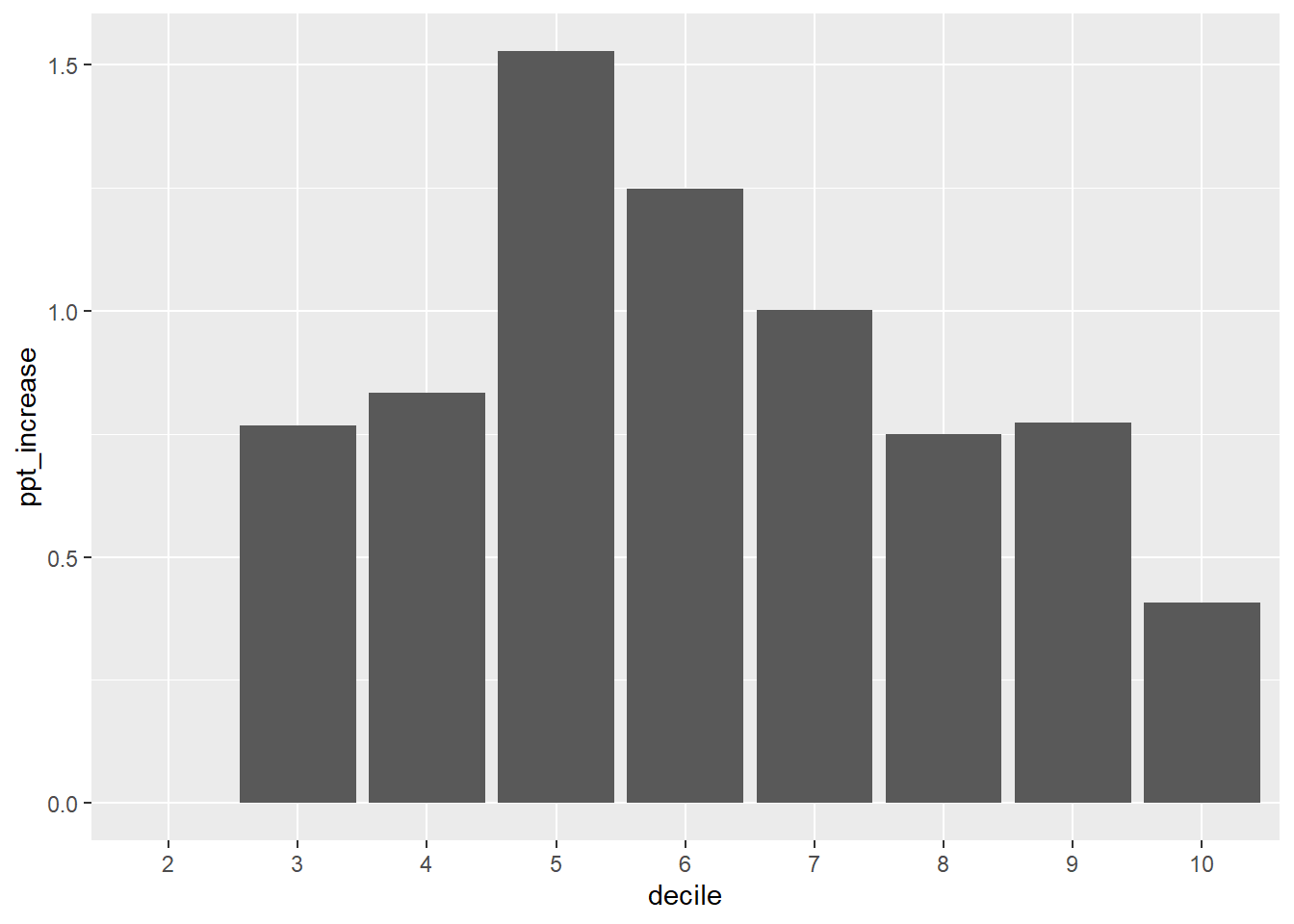This chart and the underlying data are a reflection of the assumptions in the project function. In particular, the project function uses a particular forecast of the wage price index to uprate the salary column in the sample file. This differs from the assumptions in the 2017 Budget.

Budget_wage_series <-
data.table(fy_year = c("2017-18", "2018-19", "2019-20", "2020-21"),
r = c(0.025, 0.03, 0.035, 0.0375))

kable(Budget_wage_series)
fy_year r
2017-18 0.0250
2018-19 0.0300
2019-20 0.0350
2020-21 0.0375

The project function expects wages to grow by 8% over the period 2016-17 to 2020-21, whereas by the forecast in the Budget this number would be 13%. Whatever the merits of each forecast, the project function allows you to specify a particular wage series in the future through the argument wage.series =. We can then repeat the analysis above using those estimates:

sample_file_1617 <- project(sample_file_1213,
h = 4L,
fy.year.of.sample.file = "2012-13")

sample_file_2021 <- project(sample_file_1213,
fy.year.of.sample.file = "2012-13",
h = 8L,
wage.series = Budget_wage_series)

sample_file_1617[, tax_paid := income_tax(Taxable_Income,
.dots.ATO = copy(sample_file_1617),
fy.year = "2016-17")]
sample_file_1617[, avg_tax := tax_paid / Taxable_Income]
sample_file_2021[, tax_paid := income_tax(Taxable_Income,
.dots.ATO = copy(sample_file_2021),
fy.year = "2019-20")]
sample_file_2021[, avg_tax := tax_paid / Taxable_Income]

avg_tax_by_decile_1617 <-
sample_file_1617 %>%
.[, .(avg_tax = mean(avg_tax)),
keyby = .(decile = weighted_ntile(Taxable_Income, n = 10))]

avg_tax_by_decile_2021 <-
sample_file_2021 %>%
.[, .(avg_tax = mean(avg_tax)),
keyby = .(decile = weighted_ntile(Taxable_Income, n = 10))]

difference_2021_Budget <-
avg_tax_by_decile_1617[avg_tax_by_decile_2021] %>%
.[decile > 1] %>%
.[, ppt_increase := 100*(i.avg_tax - avg_tax)]

difference_2021_Budget %>%
copy %>%
.[, decile := factor(decile)] %>%
ggplot(aes(x = decile, y = ppt_increase)) +
geom_col()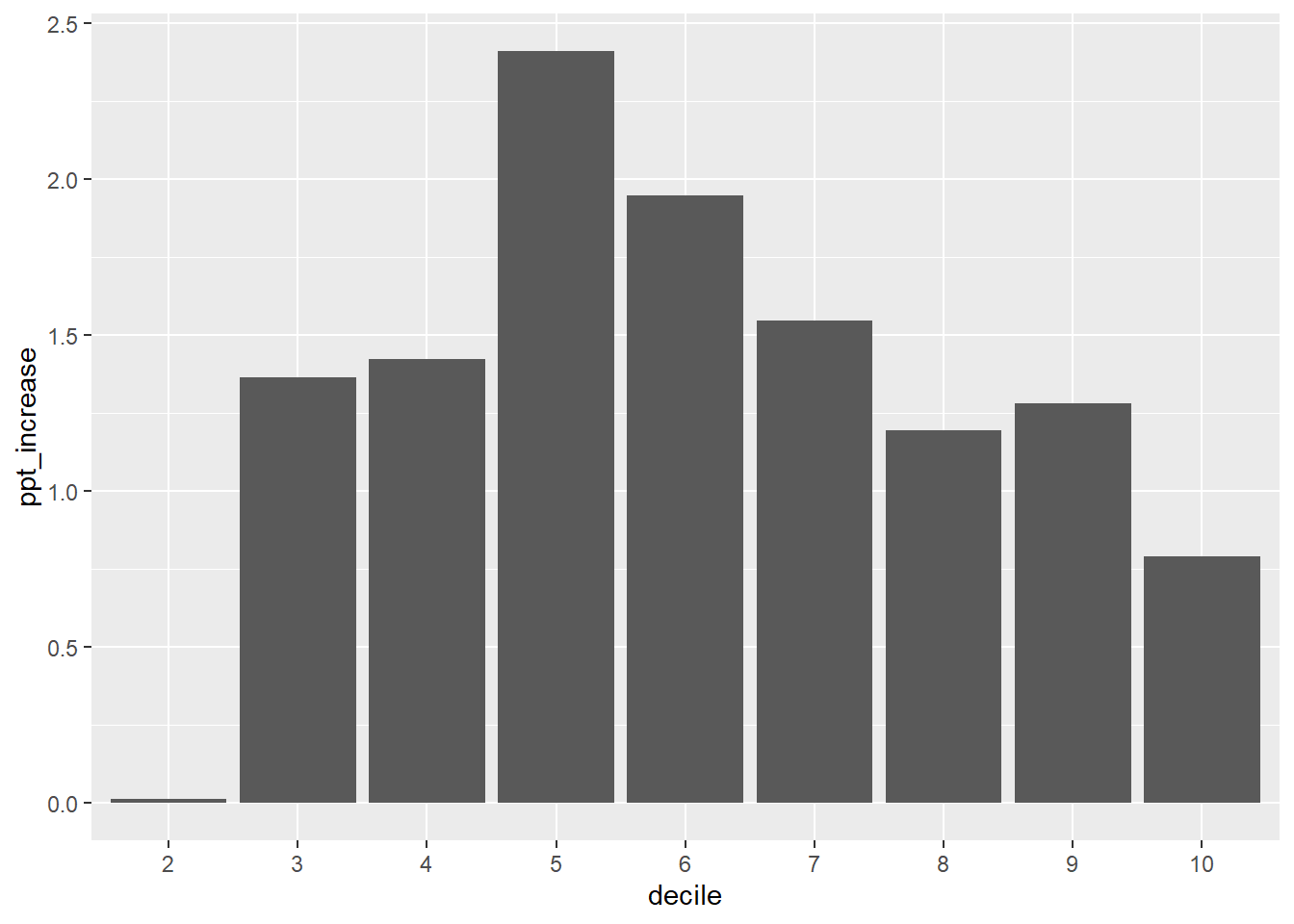Through some intermediate calculations, we can obtain the sentence that was used in the op-ed:

middle_income_avg_inc <-
difference_2021_Budget %>%
.[decile %between% c(3, 7)] %$% range(round(ppt_increase, 1)) sample_file_1617[, percentile := weighted_ntile(Taxable_Income, n = 100)] stopifnot(56 %in% sample_file_1617[Taxable_Income %between% c(49500, 50500)][["percentile"]]) avg_tax_rate_2017_50k <- sample_file_1617[percentile == 56] %$%
mean(avg_tax) %>%
round(3)

avg_tax_rate_2021_50k <-
sample_file_2021 %>%
.[, percentile := weighted_ntile(Taxable_Income, n = 100)] %>%
.[percentile == 56] %$% mean(avg_tax) %>% round(3) Middle-income earners are particularly hurt by bracket creep. Based on the wages growth projected in the 2016 budget, the average tax rates for people in middle-income groups will increase by between 1.4 and 2.4 percentage points by 2021. For example, a person earning$50,000 a year will go from paying an average tax rate of 17 per cent in 2017 to 18.9 per cent in 2021.

### Income tax with changes to rates

We can also use the package to estimate the revenue difference under changes to the marginal tax rates. The following function accepts two arguments: the bracket number to modified, and a rate increase to that bracket. The function returns the change in revenue (as a loss).

tax_delta <- function(bracket_number, rate_increase = -0.01) {
current_tax <-
sample_file_2021[, .(tax = sum(tax_paid),
WEIGHT = WEIGHT)] %$% sum(tax * WEIGHT) orig_rates <- c(0, 0.19, 0.325, 0.37, 0.45) new_rates <- orig_rates new_rates[bracket_number] <- new_rates[bracket_number] + rate_increase # rebate_income is an internal function .ri <- grattan:::rebate_income new_tax <- sample_file_2021 %>% # memory is tight on e.g. Travis-CI selector(cols = c("age_range", "Net_rent_amt", "Rep_frng_ben_amt", "Spouse_adjusted_taxable_inc", "Net_fincl_invstmt_lss_amt", "Rptbl_Empr_spr_cont_amt", "Taxable_Income", "WEIGHT", "tax_paid")) %>% .[, base_tax. := IncomeTax(Taxable_Income, thresholds = c(0, 18200, 37000, 87000, 180e3), rates = new_rates)] %>% .[, medicare_levy. := medicare_levy(income = Taxable_Income, fy.year = "2019-20", Spouse_income = Spouse_adjusted_taxable_inc, sapto.eligible = (age_range <= 1), family_status = if_else(Spouse_adjusted_taxable_inc > 0, "family", "individual"))] %>% .[, lito. := lito(Taxable_Income, max_lito = 445, lito_taper = 0.015, min_bracket = 37000)] %>% .[, rebate_income := .ri(Taxable_Income, Rptbl_Empr_spr_cont_amt = Rptbl_Empr_spr_cont_amt, Net_fincl_invstmt_lss_amt = Net_fincl_invstmt_lss_amt, Net_rent_amt = Net_rent_amt, Rep_frng_ben_amt = Rep_frng_ben_amt)] %>% .[, sapto. := sapto(rebate_income, fy.year = "2019-20", sapto.eligible = (age_range <= 1))] %>% .[, tax_payable := pmaxC(base_tax. - lito. - sapto., 0) + medicare_levy.] %>% .[, .(tax = sum(tax_payable), WEIGHT = WEIGHT)] %$%
sum(tax * WEIGHT)

current_tax - new_tax
}

which leads to the sentence and table in the op-ed:

For example, if the government was to reduce the tax rate only in the middle (37,000-87,000) bracket from 32.5% to 30%, the promised $7.8 billion surplus in 2021 would all but be swallowed up by the 6.6 bn revenue loss. data.table(tax_bracket = c("<18,200", "18,200-37,000", "37,000-87,000", "87,000-180,000", "180,000+"), budget_impact = c(NA, round(vapply(2:5, tax_delta, FUN.VALUE = double(1)) / 1e9, 2))) %>% kable tax_bracket budget_impact <18,200 NA 18,200-37,000 1.20 37,000-87,000 2.19 87,000-180,000 0.73 180,000+ 0.24 # Companion to the 2013-14 sample file ## Prologue This vignette is a mirror of a small book prepared internally by Grattan Institute. The goal is to demonstrate how to perform simple analysis and create common charts. You will need the taxstats package available via devtools::install_github('hughparsonage/taxstats'). options("scipen" = 99) opts_chunk$set(fig.width = 9,
fig.height = 6.5,
warn = FALSE)
FY.YEAR <- "2013-14"
wsum <- function(x, w = 1){
sum((x) * w)
}
grattan_dollar <- function (x, digits = 0) {
#
nsmall <- digits
commaz <- format(abs(x), nsmall = nsmall, trim = TRUE, big.mark = ",",
scientific = FALSE, digits = 1L)

if_else(x < 0,
paste0("\U2212","$", commaz), paste0("$", commaz))
}
sample_file <- sample_files_all[fy.year == FY.YEAR]
sample_file <- merge(sample_file, age_range_decoder, by = "age_range")
PREV.FY.YEAR <- yr2fy(fy2yr(FY.YEAR) - 1)
sample_file_prev <- sample_files_all[fy.year == PREV.FY.YEAR]
sample_file_prev <- merge(sample_file_prev, age_range_decoder, by = "age_range")
impute_ages <- function(DT) {
age_range_description <- NULL
DT[grepl("to", age_range_description), min_age := as.integer(sub("^([0-9]+).*$", "\\1", age_range_description))] DT[grepl("70", age_range_description), min_age := 70L] DT[, min_age := coalesce(min_age, 15L)] DT[, max_age := min_age + 5L] if (requireNamespace("withr", quietly = TRUE)) { withr::with_seed(48031, { DT[, age_imp := runif(.N, min_age, max_age), by = .(min_age)] }) } else { DT[, age_imp := runif(.N, min_age, max_age), by = .(min_age)] } DT[, c("min_age", "max_age") := NULL] DT[] } impute_ages(sample_file) #> age_range fy.year Ind Gender Birth_year Occ_code Marital_status #> 1: 0 2013-14 43087 0 NA 9 NA #> 2: 0 2013-14 208017 0 NA 0 NA #> 3: 0 2013-14 193396 1 NA 0 NA #> 4: 0 2013-14 187829 0 NA 0 NA #> 5: 0 2013-14 86889 0 NA 2 NA #> --- #> 258770: 11 2013-14 110282 1 NA 9 NA #> 258771: 11 2013-14 109792 0 NA 8 NA #> 258772: 11 2013-14 118192 1 NA 6 NA #> 258773: 11 2013-14 236180 1 NA 0 NA #> 258774: 11 2013-14 142336 1 NA 6 NA #> Region Lodgment_method HECS_accum_ind PHI_Ind Sw_amt Alow_ben_amt #> 1: 122 A NA 0 3473 0 #> 2: 503 A NA 1 0 0 #> 3: 309 A NA 0 0 0 #> 4: 311 A NA 1 0 0 #> 5: 208 S NA 1 20612 0 #> --- #> 258770: 119 S NA 1 11843 0 #> 258771: 310 A NA 0 25726 0 #> 258772: 116 S NA 0 26292 0 #> 258773: 309 A NA 1 0 0 #> 258774: 127 A NA 0 5132 0 #> Grs_int_amt Aust_govt_pnsn_allw_amt Frk_Div_amt Net_rent_amt #> 1: 821 16106 0 0 #> 2: 299 7190 0 0 #> 3: 23042 0 0 0 #> 4: 4333 0 0 0 #> 5: 914 0 0 10282 #> --- #> 258770: 18 0 0 0 #> 258771: 0 0 0 0 #> 258772: 2 0 0 0 #> 258773: 12 0 0 0 #> 258774: 2 0 0 0 #> Gross_rent_amt Other_rent_ded_amt Rent_int_ded_amt Rent_cap_wks_amt #> 1: 0 0 0 0 #> 2: 0 0 0 0 #> 3: 0 0 0 0 #> 4: 0 0 0 0 #> 5: 16356 6074 0 0 #> --- #> 258770: 0 0 0 0 #> 258771: 0 0 0 0 #> 258772: 0 0 0 0 #> 258773: 0 0 0 0 #> 258774: 0 0 0 0 #> Net_PP_BI_amt Net_NPP_BI_amt Total_PP_BI_amt Total_NPP_BI_amt #> 1: 0 0 0 0 #> 2: 0 256 0 256 #> 3: 0 0 0 0 #> 4: -13235 0 0 0 #> 5: 0 0 0 0 #> --- #> 258770: 0 0 0 0 #> 258771: 0 0 0 0 #> 258772: 0 0 0 0 #> 258773: 0 0 0 0 #> 258774: 0 0 0 0 #> Total_PP_BE_amt Total_NPP_BE_amt Net_CG_amt Tot_CY_CG_amt Net_PT_PP_dsn #> 1: 0 0 0 0 0 #> 2: 0 0 0 0 0 #> 3: 0 0 0 0 0 #> 4: 13235 0 0 0 0 #> 5: 0 0 0 0 0 #> --- #> 258770: 0 0 0 0 0 #> 258771: 0 0 0 0 0 #> 258772: 0 0 0 0 0 #> 258773: 0 0 0 0 0 #> 258774: 0 0 0 0 0 #> Net_PT_NPP_dsn Othr_pnsn_amt Other_foreign_inc_amt Other_inc_amt #> 1: 0 NA 0 0 #> 2: 0 NA 0 0 #> 3: 987 NA 1015 4994 #> 4: 0 NA 0 8926 #> 5: 0 NA 0 0 #> --- #> 258770: 0 NA 0 0 #> 258771: 0 NA 0 0 #> 258772: 0 NA 0 0 #> 258773: 0 NA 0 0 #> 258774: 0 NA 0 0 #> Tot_inc_amt WRE_car_amt WRE_trvl_amt WRE_uniform_amt WRE_self_amt #> 1: 23062 0 0 0 0 #> 2: 43728 0 0 0 0 #> 3: 30038 0 0 0 0 #> 4: 24 0 0 0 0 #> 5: 31808 488 0 603 0 #> --- #> 258770: 11861 0 0 88 0 #> 258771: 25726 160 0 217 0 #> 258772: 26294 1211 0 127 0 #> 258773: 12 0 0 0 0 #> 258774: 5134 0 0 0 0 #> WRE_other_amt Int_Div_ded_amt Gift_amt Non_emp_spr_amt #> 1: 277 NA 0 0 #> 2: 0 NA 583 0 #> 3: 0 NA 0 0 #> 4: 0 NA 0 0 #> 5: 971 NA 494 0 #> --- #> 258770: 146 NA 0 0 #> 258771: 409 NA 0 0 #> 258772: 121 NA 19 0 #> 258773: 0 NA 0 0 #> 258774: 0 NA 0 0 #> Cost_tax_affairs_amt Other_Ded_amt Tot_ded_amt PP_loss_claimed #> 1: 0 0 277 0 #> 2: 187 0 770 0 #> 3: 354 0 1443 0 #> 4: 0 0 0 0 #> 5: 0 0 2632 0 #> --- #> 258770: 0 0 234 0 #> 258771: 0 0 786 0 #> 258772: 0 0 1478 0 #> 258773: 0 0 0 0 #> 258774: 0 0 0 0 #> NPP_loss_claimed Rep_frng_ben_amt Med_Exp_TO_amt Taxable_Income #> 1: 0 0 0 22785 #> 2: 0 0 0 42958 #> 3: 0 0 0 28595 #> 4: 0 0 0 24 #> 5: 0 0 779 29176 #> --- #> 258770: 0 0 0 11627 #> 258771: 0 0 0 24940 #> 258772: 0 0 0 24816 #> 258773: 0 0 0 12 #> 258774: 0 0 0 5134 #> Taxed_othr_pnsn_amt Untaxed_othr_pnsn_amt Partner_status Div_Ded_amt #> 1: 0 2662 0 0 #> 2: 0 35983 1 0 #> 3: 0 0 0 0 #> 4: 0 0 1 0 #> 5: 0 0 1 0 #> --- #> 258770: 0 0 0 0 #> 258771: 0 0 0 0 #> 258772: 0 0 0 0 #> 258773: 0 0 0 0 #> 258774: 0 0 0 0 #> Intrst_Ded_amt ETP_txbl_amt Unfranked_Div_amt Dividends_franking_cr_amt #> 1: 0 0 0 0 #> 2: 0 0 0 0 #> 3: 1089 0 0 0 #> 4: 0 0 0 0 #> 5: 76 0 0 0 #> --- #> 258770: 0 0 0 0 #> 258771: 0 0 0 0 #> 258772: 0 0 0 0 #> 258773: 0 0 0 0 #> 258774: 0 0 0 0 #> Net_farm_management_amt Asbl_forgn_source_incm_amt #> 1: 0 0 #> 2: 0 0 #> 3: 0 1015 #> 4: 0 0 #> 5: 0 0 #> --- #> 258770: 0 0 #> 258771: 0 0 #> 258772: 0 0 #> 258773: 0 0 #> 258774: 0 0 #> Spouse_adjusted_taxable_inc Net_fincl_invstmt_lss_amt #> 1: 0 0 #> 2: 3228 0 #> 3: 0 0 #> 4: 25353 0 #> 5: 34443 0 #> --- #> 258770: 0 0 #> 258771: 0 0 #> 258772: 0 0 #> 258773: 0 0 #> 258774: 0 0 #> Rptbl_Empr_spr_cont_amt Cr_PAYG_ITI_amt TFN_amts_wheld_gr_intst_amt #> 1: 0 0 0 #> 2: 0 0 0 #> 3: 0 0 0 #> 4: 0 0 0 #> 5: 0 0 0 #> --- #> 258770: 0 0 0 #> 258771: 0 0 0 #> 258772: 0 0 0 #> 258773: 0 0 0 #> 258774: 0 0 0 #> TFN_amts_wheld_divs_amt Hrs_to_prepare_BPI_cnt Help_debt #> 1: 0 0 0 #> 2: 0 0 0 #> 3: 0 0 0 #> 4: 0 0 0 #> 5: 0 0 0 #> --- #> 258770: 0 0 0 #> 258771: 0 0 0 #> 258772: 0 0 0 #> 258773: 0 0 0 #> 258774: 0 0 0 #> MCS_Emplr_Contr MCS_Prsnl_Contr MCS_Othr_Contr MCS_Ttl_Acnt_Bal WEIGHT #> 1: 236 0 0 137940 50 #> 2: 0 0 0 18259 50 #> 3: 0 0 0 0 50 #> 4: 0 0 0 0 50 #> 5: 1856 0 356 651702 50 #> --- #> 258770: 915 0 0 762 50 #> 258771: 2371 0 49 2450 50 #> 258772: 2146 0 289 4163 50 #> 258773: 0 0 0 0 50 #> 258774: 0 0 0 0 50 #> age_range_description age_imp #> 1: 70 and over 74.74251 #> 2: 70 and over 74.58943 #> 3: 70 and over 74.21550 #> 4: 70 and over 73.97913 #> 5: 70 and over 70.69816 #> --- #> 258770: under 20 15.24481 #> 258771: under 20 17.64674 #> 258772: under 20 17.04917 #> 258773: under 20 18.14840 #> 258774: under 20 19.83487 sample_file[, Tax_Bracket := cut(Taxable_Income, breaks = c(-Inf, 18200, 37e3, 80e3, 180e3, Inf), include.lowest = TRUE, labels = c("$0-$18,200", "$18,201-$37,000", "37,001-$80,000",
"$80,001-$180,000",
"$180,000+"))] texNum <- function(number, sig.figs = 3L, dollar = FALSE, pre.phrase = NULL, .suffix = NULL){ orig.number <- number stopifnot(is.numeric(number), length(number) == 1L) is.negative <- number < 0 number <- abs(number) if (number == 0){ warning("Returning 0") return(0) } else { if (is.null(.suffix)){ n.digits <- ceiling(log10(number)) suffix <- NULL suffix_val <- 1 if (n.digits < sig.figs){ prefix <- signif(x = number, digits = sig.figs) } else { if (n.digits <= 6) { prefix_val <- round(number, sig.figs - n.digits - 1) prefix <- prettyNum(prefix_val, big.mark = ",", scientific = FALSE) } else { # Want to show only the number / 10^(multiple of 3) then the suffix multiplier suffix_val <- 10 ^ (3 * ((n.digits %/% 3))) prefix_val <- signif(number/suffix_val, digits = sig.figs) prefix <- prefix_val if (suffix_val <= 10^12){ switch(log10(suffix_val) / 3 - 1, suffix <- "~million", suffix <- "~billion", suffix <- "~trillion") } else { prefix <- signif(number / 10^12, digits = sig.figs) suffix <- "~trillion" } } } } else { stopifnot(.suffix %in% c("million", "billion", "trillion")) switch(.suffix, "million" = { prefix <- signif(number / 10^6, digits = sig.figs) suffix <- "~million" suffix_val <- 10^6 }, "billion" = { prefix <- signif(number / 10^9, digits = sig.figs) suffix <- "~billion" suffix_val <- 10^9 }, "trillion" = { prefix <- signif(number / 10^12, digits = sig.figs) suffix <- "~trillion" suffix_val <- 10^12 }) prefix_val <- prefix } if (dollar){ out <- paste0("\\$", prefix, suffix)
} else {
out <- paste0(prefix, suffix)
}

if (is.negative){
# General LaTeX
out <- paste0("\$$-\$$", out)
}
# is the displayed number larger than the original?
if (!is.null(pre.phrase)){
out_larger <- prefix_val * suffix_val > orig.number

if (out_larger) {
out <- paste(pre.phrase, out, sep = if(grepl("~$", pre.phrase)) "" else " ") } else { if (!isTRUE(all.equal(prefix_val * suffix_val, orig.number, tolerance = .Machine$double.eps)))
out <- paste(pre.phrase, out, sep = if(grepl("~$", pre.phrase)) "" else " ") } } return(out) } } sample_file <- as.data.table(sample_file) There were 12.9~million taxpayers in 2013-14 in Australia. Of those, 180,000 had zero taxable income (or a taxable loss). (… and so these ‘’taxpayers’’ naturally paid no tax. Nor did the 2.5~million individuals below the tax-free threshold. For this vignette, a taxpayer is anyone who lodged a tax return, regardless of their tax liability). tx_inc_q <- function(q){ quantile(sample_file$Taxable_Income, probs = q)
}

my_labs <- grattan_dollar(tx_inc_q((0:10)/10))
my_labs[seq(2, 10, 2)] <- paste0("\n", my_labs[seq(2, 10, 2)])

dens <- density(sample_file[Taxable_Income < tx_inc_q(0.95)]$Taxable_Income) DF <- with(dens, data.frame(x, y)) sample_file %>% lazy_dt %>% mutate(Taxable_Income_decile = ntile(Taxable_Income, 10)) %>% filter(between(Taxable_Income, 0, tx_inc_q(0.95))) %>% as.data.table %>% ggplot(aes(x = Taxable_Income)) + geom_density() + scale_fill_viridis(discrete = TRUE) + scale_x_continuous("Taxable Income deciles", labels = c(my_labs, grattan_dollar(tx_inc_q(0.95))), # limits = c(0, tx_inc_q(0.95)), breaks = c(tx_inc_q((0:10)/10), tx_inc_q(0.95))) + scale_y_continuous(expand = c(0,0)) + theme(legend.position = "none", axis.line.y = element_blank(), axis.text.y = element_blank(), axis.title.y = element_blank())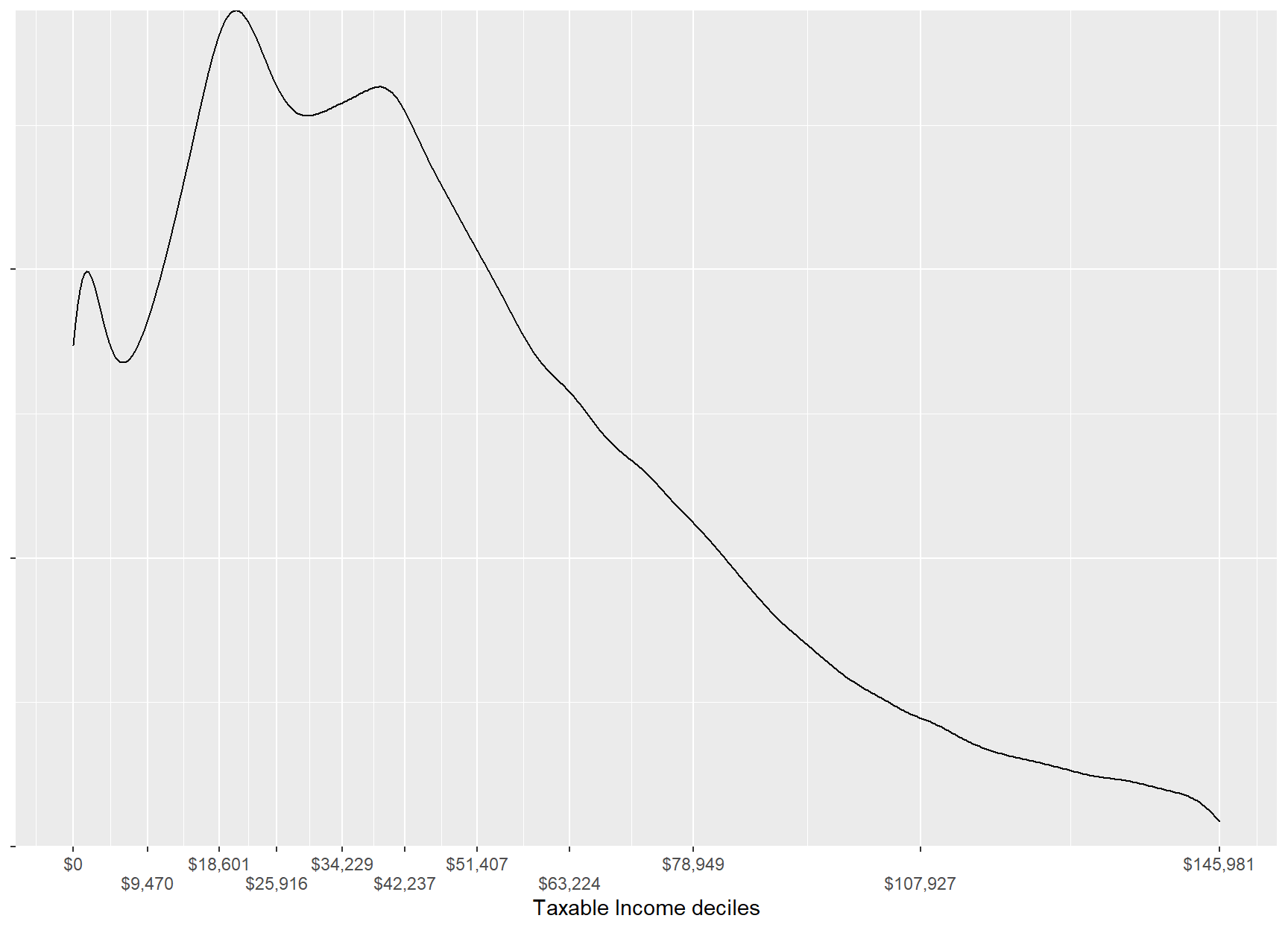DF %>% mutate(Taxable_Income_decile = cut(x, breaks = quantile(sample_file$Taxable_Income,
probs = c(0:10)/10),
right = TRUE,
include.lowest = TRUE)) %>%
filter(between(x, -1, tx_inc_q(0.95) * 1.05)) %>%
{
ggplot(., aes(x = x, y = y)) +
geom_area(color = "black", size = 1.45) +
geom_area(aes(x = x, y = y,
group = Taxable_Income_decile,
fill = factor(Taxable_Income_decile),
color = factor(Taxable_Income_decile))) +
scale_color_viridis(discrete = TRUE) +
scale_fill_viridis(discrete = TRUE) +
scale_x_continuous("Taxable Income deciles",
labels = c(my_labs, grattan_dollar(tx_inc_q(0.95))),
expand = c(0,0),
# limits = c(-1, tx_inc_q(0.95)*1.05),
breaks = c(tx_inc_q((0:10)/10), tx_inc_q(0.95))) +
scale_y_continuous(expand = c(0,0), limits = c(0, max(.$y) * 1.05)) + theme(legend.position = "none", axis.line.y = element_blank(), axis.text.y = element_blank(), axis.title.y = element_blank())+ annotate("text", x = tx_inc_q(0.925), y = 2 * max(.$y[.$x > tx_inc_q(0.925)]), size = 10/(14/5), label = paste0("5% of taxpayers\nhad incomes\ngreater than\n", grattan_dollar(tx_inc_q(0.95))), hjust = 0, vjust = 0) + annotate("segment", arrow = arrow(type = "closed", length = unit(11, "pt"), angle = 20), x = tx_inc_q(0.925), y = 1.9 * max(.$y[.$x > tx_inc_q(0.925)]), size = 1, xend = tx_inc_q(0.95), yend = 1.9 * max(.$y[.$x > tx_inc_q(0.925)])) }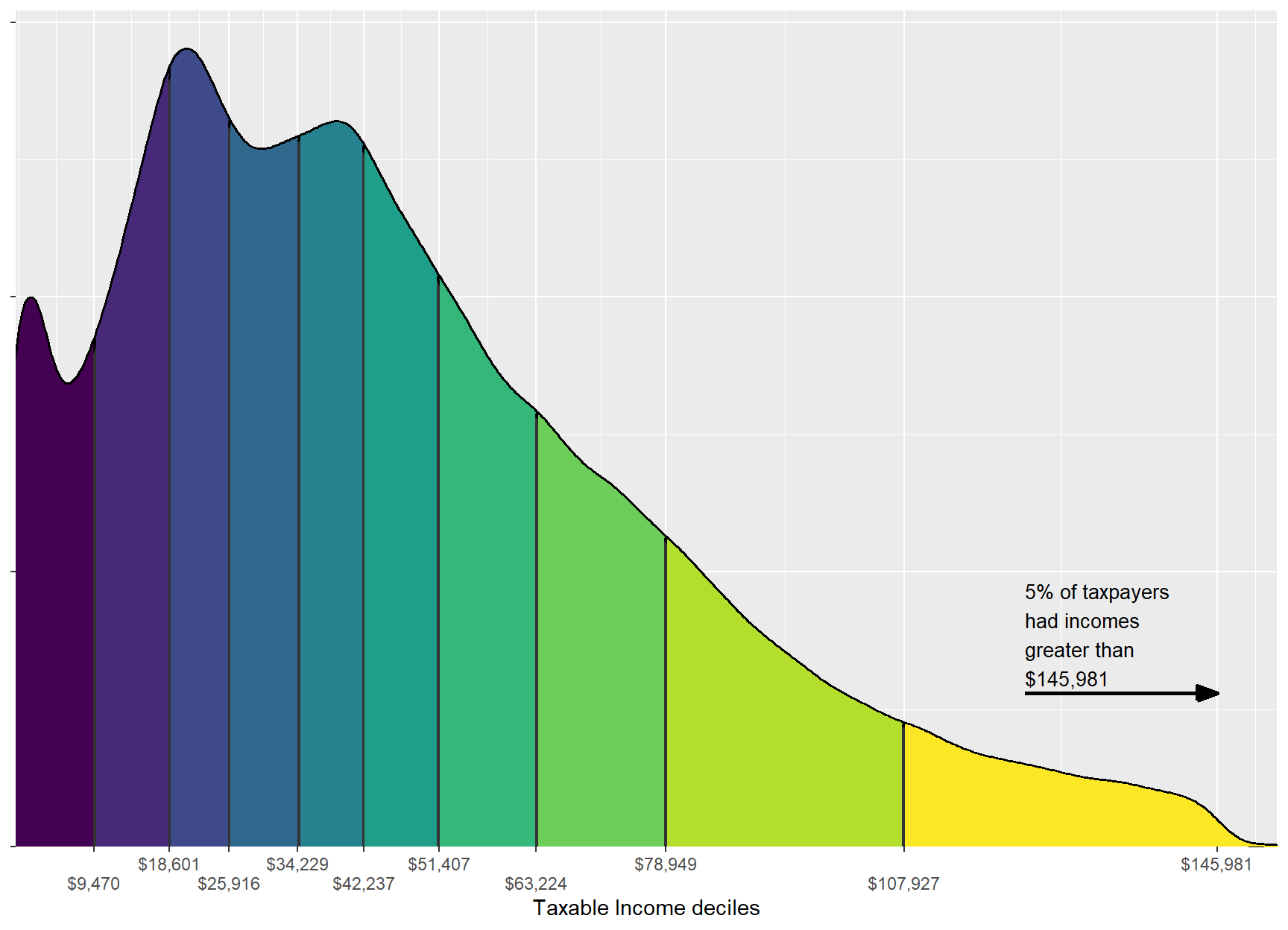sample_file <- as.data.table(sample_file) n_CGs <- sample_file %>% filter(Tot_CY_CG_amt > 0) %$%
sum(WEIGHT)
#> Warning: You are using a dplyr method on a raw data.table, which will call the
#> * data frame implementation, and is likely to be inefficient.
#> *
#> * To suppress this message, either generate a data.table translation with
#> * lazy_dt() or convert to a data frame or tibble with
#> * as.data.frame()/as_tibble().

n_CGs_prev <-
sample_file_prev %>%
filter(Tot_CY_CG_amt > 0) %$% sum(WEIGHT) #> Warning: You are using a dplyr method on a raw data.table, which will call the #> * data frame implementation, and is likely to be inefficient. #> * #> * To suppress this message, either generate a data.table translation with #> * lazy_dt() or convert to a data frame or tibble with #> * as.data.frame()/as_tibble(). tot_CG_amt <- sample_file %$%
sum(as.numeric(Tot_CY_CG_amt * WEIGHT))

tot_Net_CG_amt <-
sample_file %$% sum(as.numeric(Net_CG_amt * WEIGHT)) tax_on_CG <- sample_file %>% filter(Net_CG_amt > 0) %>% mutate(tax = income_tax(Taxable_Income, fy.year = FY.YEAR), tax_wo_CG = income_tax(pmaxC(Taxable_Income - Net_CG_amt, 0), fy.year = FY.YEAR)) %>% summarise(total = sum((tax - tax_wo_CG) * WEIGHT), avg = mean(tax - tax_wo_CG)) #> Warning: You are using a dplyr method on a raw data.table, which will call the #> * data frame implementation, and is likely to be inefficient. #> * #> * To suppress this message, either generate a data.table translation with #> * lazy_dt() or convert to a data frame or tibble with #> * as.data.frame()/as_tibble(). #> Warning: You are using a dplyr method on a raw data.table, which will call the #> * data frame implementation, and is likely to be inefficient. #> * #> * To suppress this message, either generate a data.table translation with #> * lazy_dt() or convert to a data frame or tibble with #> * as.data.frame()/as_tibble(). #> Warning: You are using a dplyr method on a raw data.table, which will call the #> * data frame implementation, and is likely to be inefficient. #> * #> * To suppress this message, either generate a data.table translation with #> * lazy_dt() or convert to a data frame or tibble with #> * as.data.frame()/as_tibble(). tax_on_CG_prev <- sample_file_prev %>% lazy_dt %>% filter(Net_CG_amt > 0) %>% mutate(tax = income_tax(Taxable_Income, fy.year = FY.YEAR), tax_wo_CG = income_tax(pmaxC(Taxable_Income - Net_CG_amt, 0), fy.year = FY.YEAR)) %>% summarise(total = sum((tax - tax_wo_CG) * WEIGHT), avg = mean(tax - tax_wo_CG))  latex_percent <- function(x) gsub("%", "\\%", percent(x), fixed = TRUE) The capital gains discount applies to assets sold after more than 12 months’ holding. There were 840,000 individuals who sold capital assets, up 9% from last year. The sale of their assets totalled$34.2~billion of which $12.9~billion comprised part of their taxable income. The tax on these capital gains totalled$4.76~billion or $7,900 per individual with capital gains tax. sample_file <- as.data.table(sample_file) probCG_by_age <- sample_file[, .(probCG = mean(Net_CG_amt > 0)), keyby = "age_range_description"] probCG_twenties <- sample_file[age_imp < 30, mean(Net_CG_amt > 0)] probCG_65p <- sample_file[age_imp >= 65, mean(Net_CG_amt > 0)] avg_marginal_rate_CG <- sample_file[Net_CG_amt > 0, .(Taxable_Income)] %>% .[, mean(income_tax(Taxable_Income + 1, fy.year = FY.YEAR) - income_tax(Taxable_Income, fy.year = FY.YEAR))] avg_marginal_rate_CG_weighted_by_CG <- sample_file[Net_CG_amt > 0, .(Net_CG_amt, Taxable_Income)] %>% .[, marginal_rate := income_tax(Taxable_Income + 1, fy.year = FY.YEAR) - income_tax(Taxable_Income, fy.year = FY.YEAR)] %>% .[, weighted.mean(marginal_rate, Net_CG_amt)] avg_marginal_rate_b4_CG <- sample_file[Net_CG_amt > 0 & age_imp >= 20] %>% .[, Taxable_Income_b4_CG := pmaxC(Taxable_Income - Net_CG_amt, 0)] %>% .[, marginal_rate_b4_CG := income_tax(Taxable_Income_b4_CG + 1, fy.year = FY.YEAR) - income_tax(Taxable_Income_b4_CG, fy.year = FY.YEAR)] %>% .[, is_in_workforce := between(age_imp, 20, 65)] %>% .[, .(avg_marginal_rate_weighted = weighted.mean(marginal_rate_b4_CG, Net_CG_amt), avg_marginal_Rate = mean(marginal_rate_b4_CG)), keyby = "is_in_workforce"] .prob_no_CGT_discounts <- sample_file[Tot_CY_CG_amt > 0, .(Net_CG_amt, Tot_CY_CG_amt)] %>% .[, apparent_discount := 1 - Net_CG_amt / Tot_CY_CG_amt] %>% .[, apparent_discount0 := apparent_discount == 0] %>% .[, apparent_discount1 := apparent_discount == 1] %>% .[, apparent_discount50 := apparent_discount %between% c(0.45, 0.55)] %>% .[, lapply(.SD, mean), .SDcols = c("apparent_discount0", "apparent_discount50", "apparent_discount1")] prop_no_CGT_discount <- .prob_no_CGT_discounts[["apparent_discount0"]] prop_100pc_CGT_discount <- .prob_no_CGT_discounts[["apparent_discount1"]] prop_50pc_CGT_discount <- .prob_no_CGT_discounts[["apparent_discount50"]] prop_no_CGT_discount_by_val <- sample_file[Tot_CY_CG_amt > 0, .(Net_CG_amt, Tot_CY_CG_amt)] %>% .[, apparent_discount := 1 - Net_CG_amt / Tot_CY_CG_amt] %$%
weighted.mean(apparent_discount == 0, Tot_CY_CG_amt)

cgt_ratio_res <- 50
sample_file %>%
selector(Tot_CY_CG_amt, Net_CG_amt, WEIGHT) %>%
.[Tot_CY_CG_amt > 0] %>%
.[, apparent_discount := Net_CG_amt / Tot_CY_CG_amt] %>%
.[, apparent_discount_round := round(apparent_discount * cgt_ratio_res) / cgt_ratio_res] %>%
.[, keyby = .(apparent_discount_round),
.(n_taxpayers = sum(WEIGHT),
n_taxpayers_by_val = sum(as.double(WEIGHT * Tot_CY_CG_amt)))] %>%
ggplot(aes(x = apparent_discount_round, y = n_taxpayers_by_val)) +
xlab("Ratio of Net capital gains to Total capital gains") +
geom_bar(stat = "identity", width = 1/cgt_ratio_res) +
theme(axis.title.y = element_blank(),
axis.ticks.y = element_blank(),
axis.text.y = element_blank())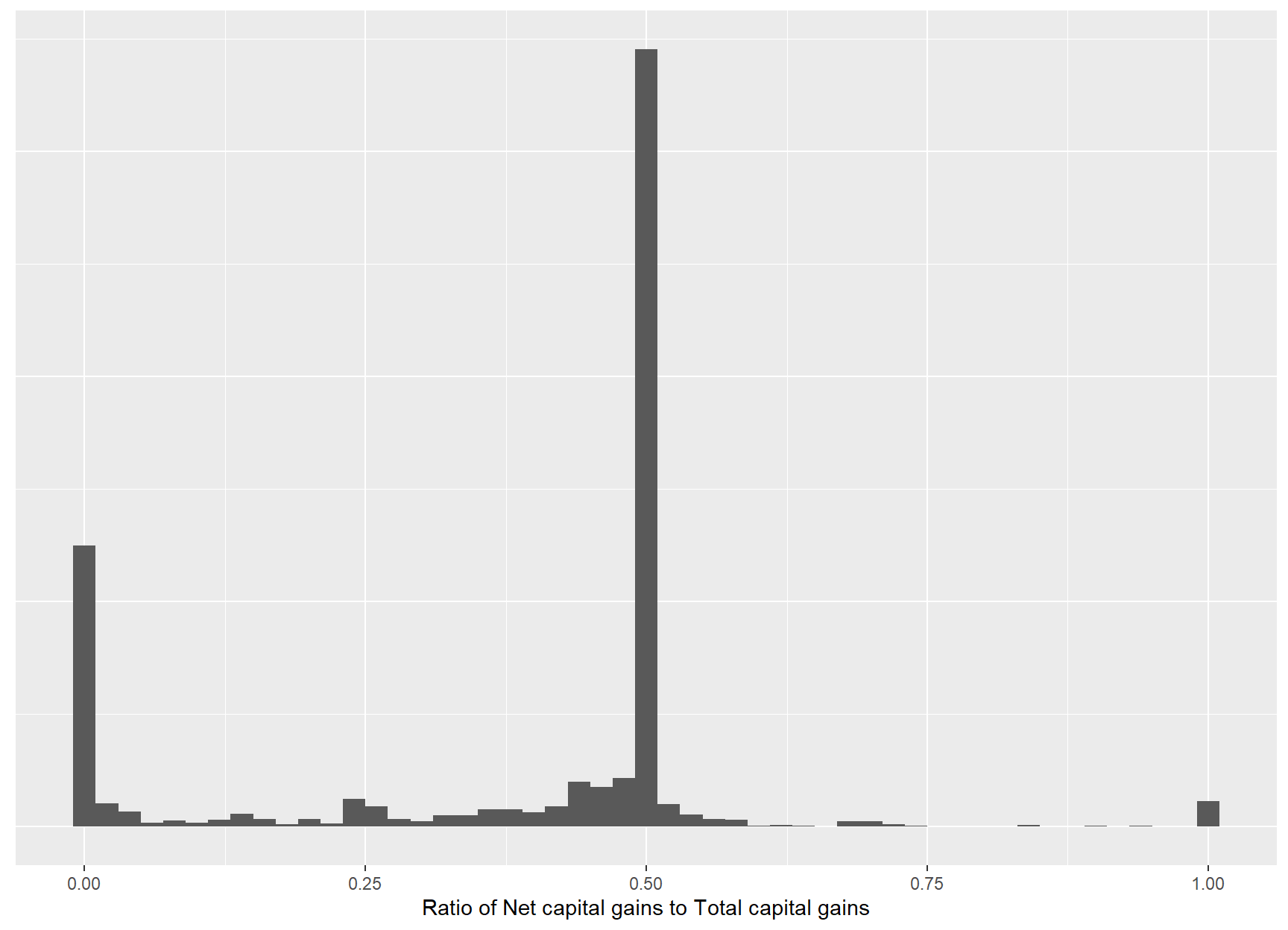Taxable capital gains are typically realized later in life. This is unsurprising: a capital gain can only be realized when one has an asset to sell. Further, the capital gains tax makes the sale of assets less attractive when incomes are high. Taxpayers in their twenties have a 1% chance of incurring capital gains tax, whereas 12% of those of retirement age have capital gains. shows that although capital gains have been more common with older taxpayers, the age skew is slightly more pronounced in 2013-14 than in previous years.

The average marginal tax rate of those with capital gains tax was 29%; however, this weights an individual with a capital gain of $1 equally as someone with a capital gain of$500,000. Weighting by the value of capital gain, the average marginal tax rate was 2 992 680%.

The net capital gains includes the CGT discount (and other discounts) applied to: $\text{Total capital gains} - \text{Total capital losses (incl. from prev. years)}$ Comparing the ratio of can shed some light on the value of the discount and the impact of capital losses on tax and tax revenue. Of those with nonzero total capital gains, 9% had no discount and 29% paid no tax (or a 100% discount). Some 45% had net capital gains of around 50% of their total gains. Weighting these numbers by the value of total capital gains, 700 697% of capital gains are taxed at the full marginal rate. shows the distribution of this ratio. The deviance from 50% is due to some gains being realized within 12 months and (more commonly) capital losses.

CG_descriptive_by_bracket <-
sample_file %>%
copy %>%
.[, tax := income_tax(Taxable_Income, fy.year = FY.YEAR)] %>%
.[, tax_wo_CG := income_tax(pmax0(Taxable_Income - Net_CG_amt), fy.year = FY.YEAR)] %>%
.[, .(n_taxpayers = sum(WEIGHT),
n_CG = sum(WEIGHT[Net_CG_amt > 0]),
val_CG = sum(as.double(Tot_CY_CG_amt * WEIGHT)),
total_CGT = sum(as.double((tax - tax_wo_CG) * WEIGHT))),
keyby = .(Tax_Bracket)]
CG_descriptive_by_bracket %>%
as.data.table %>%
# cosmetic
mutate(Taxpayers = comma(n_taxpayers),
with CG = comma(n_CG),
Total cap. gains ($) = grattan_dollar(val_CG), Total CGT ($) = grattan_dollar(total_CGT)) %>%
select(Tax bracket = Tax_Bracket,
Taxpayers, with CG, Total cap. gains ($), Total CGT ($)) %>%
kable(align = "rrrrrr")
#> Warning: You are using a dplyr method on a raw data.table, which will call the
#> * data frame implementation, and is likely to be inefficient.
#> *
#> * To suppress this message, either generate a data.table translation with
#> * lazy_dt() or convert to a data frame or tibble with
#> * as.data.frame()/as_tibble().

#> Warning: You are using a dplyr method on a raw data.table, which will call the
#> * data frame implementation, and is likely to be inefficient.
#> *
#> * To suppress this message, either generate a data.table translation with
#> * lazy_dt() or convert to a data frame or tibble with
#> * as.data.frame()/as_tibble().
Tax bracket Taxpayers with CG Total cap. gains ($$)| Total CGT ($$)
$0-$18,200 2,512,650 84,000 $2,503,663,250$0
$18,201-$37,000 3,101,900 118,300 $1,958,287,950$64,130,858
37,001-$80,000 4,808,650 185,600$4,696,316,500 $413,550,412$80,001-$180,000 2,135,400 151,350$7,373,503,900 $965,377,002$180,000+ 380,100 61,150 $17,649,661,600$3,314,621,140
sample_file %>%
ggplot(aes(x = age_imp, y = as.numeric(Net_CG_amt > 0))) +
geom_smooth(color = viridis(1), size = 1.2) +
scale_y_continuous(label = percent)
#> geom_smooth() using method = 'gam' and formula 'y ~ s(x, bs = "cs")'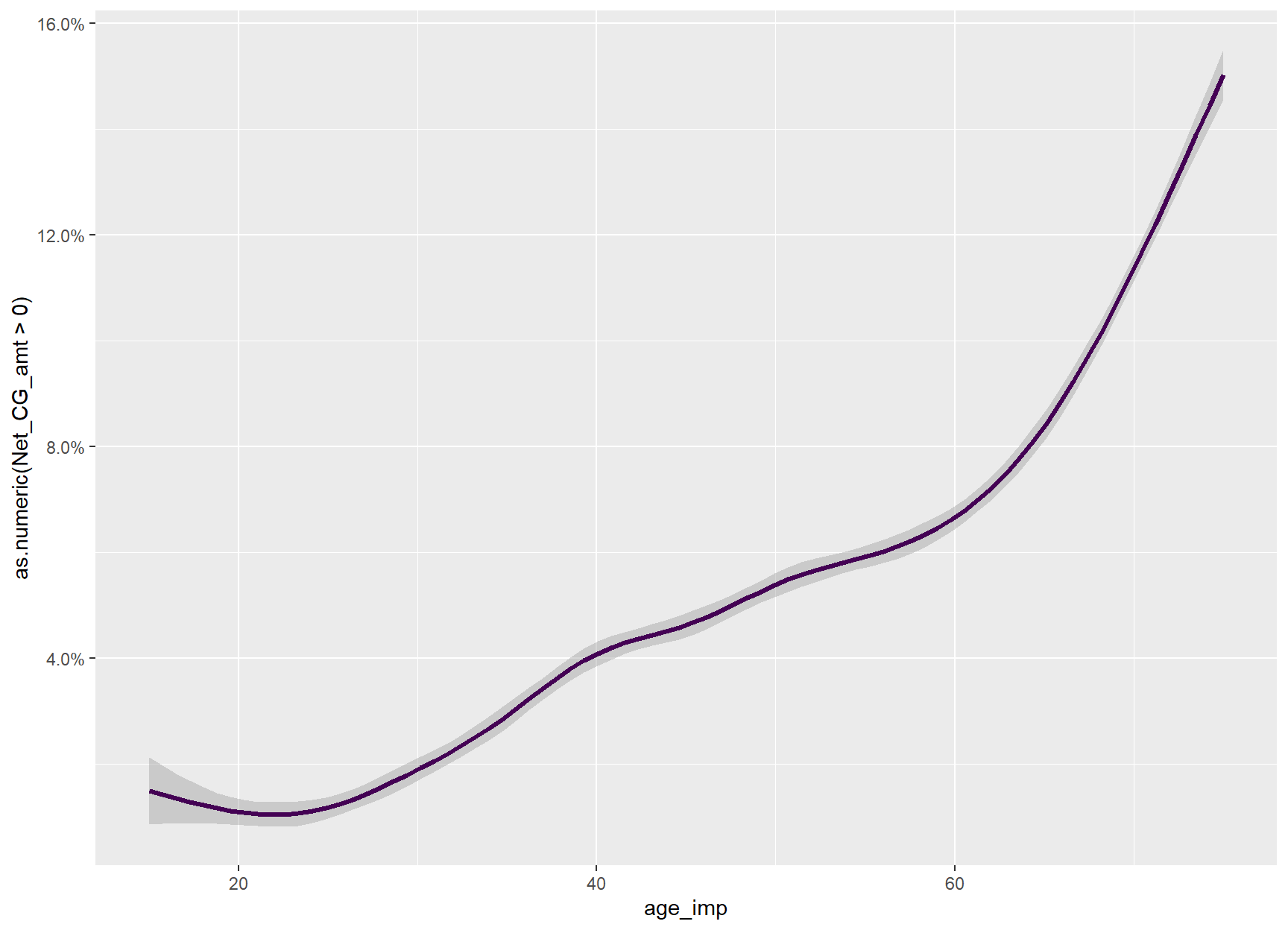# faster than ifelseing over the whole table
desc2min_age <- function(x) {
if (grepl("to", x)) {
sub("^([0-9]{2}).*$", "\\1", x) } else if (grepl("70", x)) { "70" } else { "15" } } sample_files_all %>% selector(age_range, Net_CG_amt, fy.year, shallow = TRUE) %>% merge(age_range_decoder, by = "age_range") %>% .[, min_age := desc2min_age(.BY[[1L]]), keyby = "age_range_description"] %>% .[, min_age := as.double(min_age)] %>% .[, max_age := min_age + 5] %>% .[, age_imp := rep_len(seq(from = .BY[["min_age"]], to = .BY[["max_age"]], # must be reasonably high to capture (infreq.) # capital gains events length.out = 2000), .N), keyby = c("min_age", "max_age")] %>% unique(by = c("fy.year", "age_imp")) %>% .[, last_fy := fy.year == max(fy.year)] %>% .[, Tax_year := as.character(fy.year)] %>% .[, is_CG := as.double(Net_CG_amt > 0)] %>% .[, y := fitted(gam(is_CG ~ s(age_imp, bs = "cs"), data = .SD)), keyby = "Tax_year"] %>% .[, Age := round(age_imp, 1)] %>% .[, .(y = mean(y)), keyby = .(Tax_year, Age)] %>% .[, label := if_else(Age == max(Age), Tax_year, NA_character_), keyby = "Tax_year"] %>% .[] %>% ggplot(aes(x = Age, y = y, color = Tax_year, group = Tax_year)) + scale_y_continuous(label = percent) + ggtitle("Incidence of capital gains") + scale_color_viridis("Tax year", discrete = TRUE) + geom_line(size = 1.2) + # geom_line(stat = "smooth", method = "auto", se = FALSE, size = 1.2) + geom_label_repel(aes(label = label), fill = NA, nudge_x = 1, xlim = c(75, NA), hjust = 0, vjust = 0, fontface = "bold", na.rm = TRUE) + geom_blank(aes(x = 80, y = 0)) + theme_dark() + theme(axis.title.y = element_blank())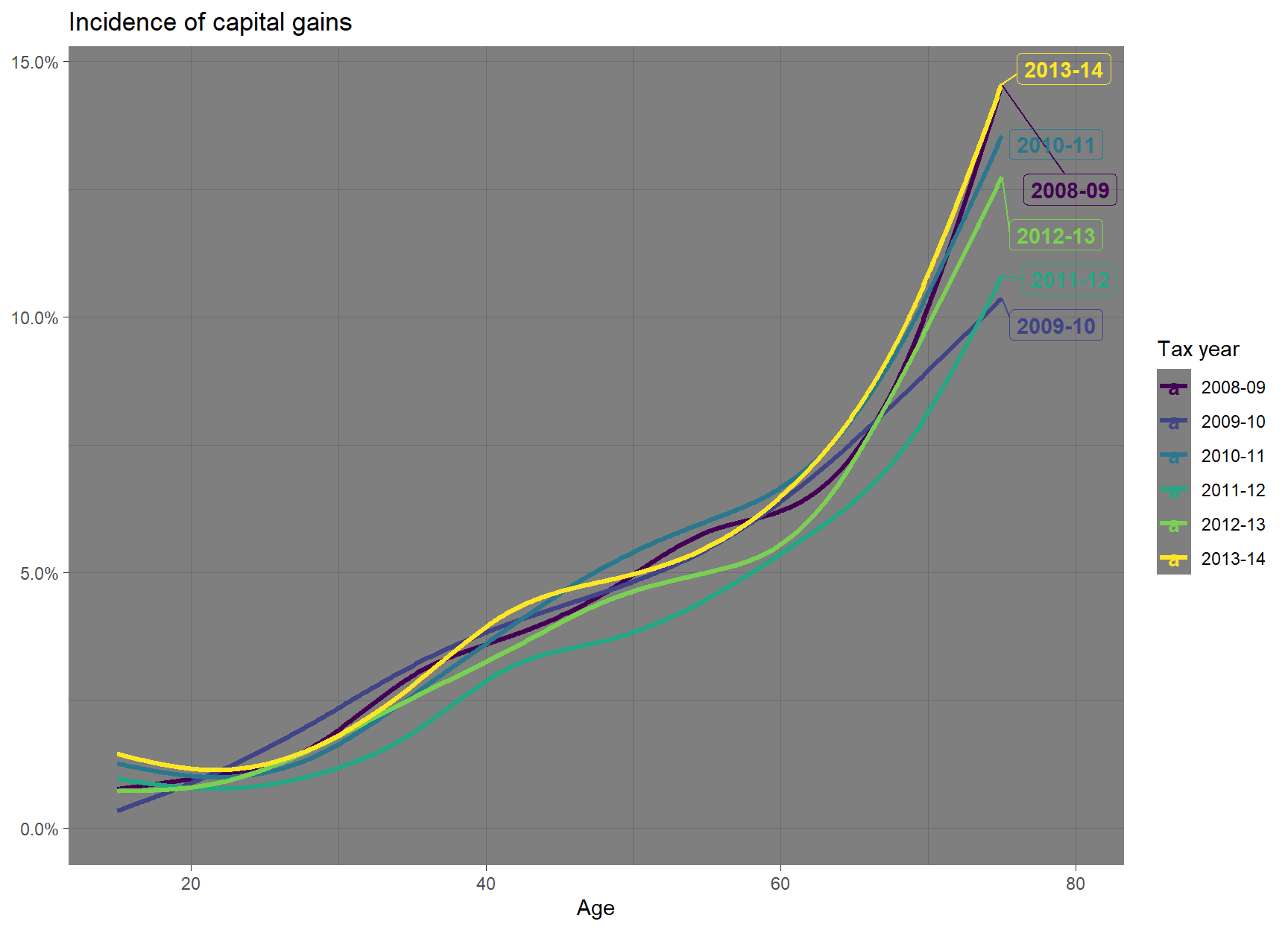with_seed <- function(s, code) { if (requireNamespace("withr", quietly = TRUE)) { eval.parent(withr::with_seed(s, code)) } else { code } } with_seed(24841, { sample_files_all %>% .[, .(age_range, Net_CG_amt, fy.year)]%>% merge(age_range_decoder, by = "age_range") %>% .[, by = .(age_range_description), min_age := if (grepl("^([0-9]{2}).*$", first(age_range_description))) {
as.numeric(gsub("^([0-9]{2}).*\$", "\\1", age_range_description))
} else {
if_else(grepl("70", age_range_description),
70,
15)
}] %>%
.[, max_age := min_age + 5] %>%
.[, age_imp := runif(.N, min_age, max_age), by = .(age_range_description)]
}) %>%
.[Net_CG_amt > 0] %>%
.[, Age := round(age_imp)] %>%

.[, .(mean_Net_CG = mean(Net_CG_amt),
sd_Net_CG = sd(Net_CG_amt)),
keyby = .(fy.year, Age)] %>%
.[, last_fy := fy.year == max(fy.year) | fy.year == max(fy.year[fy.year != max(fy.year)])] %>%
.[, label := fifelse(Age == max(Age), as.character(.BY[]), NA_character_),
keyby = .(fy.year)] %>%
.[, label.y := mean(mean_Net_CG[Age > 70])] %>%
{
ggplot(., aes(x = Age, y = mean_Net_CG, color = factor(fy.year), group = factor(fy.year))) +
scale_y_continuous(label = dollar) +
scale_color_viridis(discrete = TRUE) +
geom_line(stat = "smooth", method = "auto", se = FALSE, size = 1.2) +
scale_alpha_discrete(range = c(0.5, 1)) +
geom_text(aes(label = label, y = label.y, size = if_else(last_fy %in% c("2012-13", "2013-14"), 2, 1),
nudge_x = if_else(last_fy, 1, 0)),
hjust = 0,
vjust = 0,
fontface = "bold",
na.rm = TRUE) +
scale_x_continuous(expand = c(0,0)) +
theme_dark() +
annotate("blank",
x = 85, y = 0) +
theme(axis.title.y = element_blank(),
plot.margin = unit(c(0,0,5,0), "pt"))
}
#> Warning: Using alpha for a discrete variable is not advised.
#> Warning: Ignoring unknown aesthetics: nudge_x
#> Warning: Ignoring unknown aesthetics: x, y
#> geom_smooth() using method = 'loess' and formula 'y ~ x'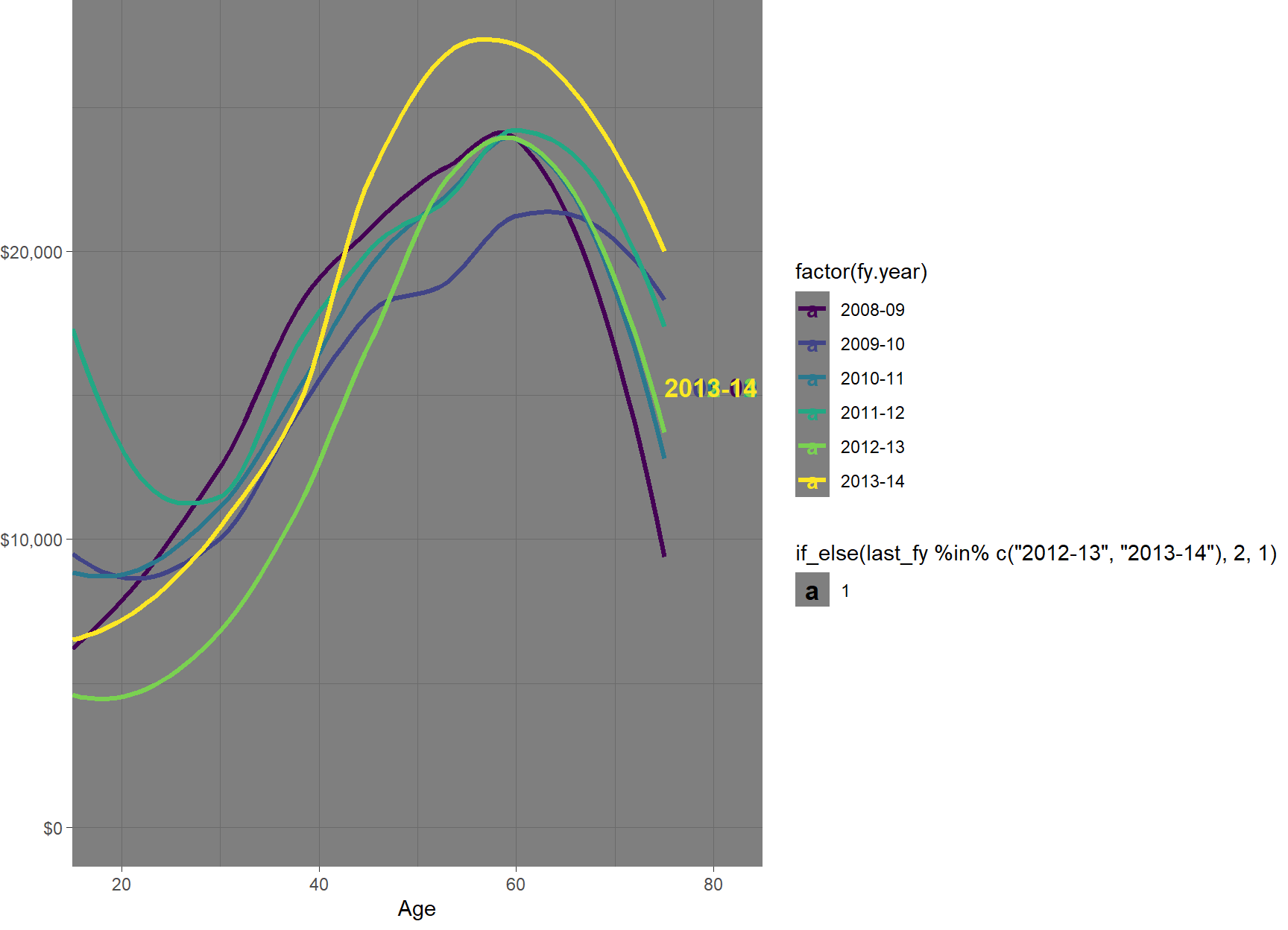sample_file %>%
mutate(Tot_inc_amt_noCG = Tot_inc_amt - Net_CG_amt,
Taxable_Income_noCG = pmaxC(Tot_inc_amt_noCG - Tot_ded_amt - NPP_loss_claimed - PP_loss_claimed, 0)) %>%
mutate(Taxable_Income_noCG_decile = ntile(Taxable_Income_noCG, 10)) %>%
filter(Taxable_Income_noCG_decile %in% c(1, 5, 10)) %>%
filter(Net_CG_amt > 0) %>%
rename(Age = age_imp) %>%
mutate(Taxable Income\n(excl CG) decile = factor(Taxable_Income_noCG_decile)) %>%
ggplot(aes(x = Age, fill = Taxable Income\n(excl CG) decile)) +
geom_density(alpha = 0.7) +
theme(legend.position = "right")
#> Warning: You are using a dplyr method on a raw data.table, which will call the
#> * data frame implementation, and is likely to be inefficient.
#> *
#> * To suppress this message, either generate a data.table translation with
#> * lazy_dt() or convert to a data frame or tibble with
#> * as.data.frame()/as_tibble().

#> Warning: You are using a dplyr method on a raw data.table, which will call the
#> * data frame implementation, and is likely to be inefficient.
#> *
#> * To suppress this message, either generate a data.table translation with
#> * lazy_dt() or convert to a data frame or tibble with
#> * as.data.frame()/as_tibble().

#> Warning: You are using a dplyr method on a raw data.table, which will call the
#> * data frame implementation, and is likely to be inefficient.
#> *
#> * To suppress this message, either generate a data.table translation with
#> * lazy_dt() or convert to a data frame or tibble with
#> * as.data.frame()/as_tibble().

#> Warning: You are using a dplyr method on a raw data.table, which will call the
#> * data frame implementation, and is likely to be inefficient.
#> *
#> * To suppress this message, either generate a data.table translation with
#> * lazy_dt() or convert to a data frame or tibble with
#> * as.data.frame()/as_tibble().

#> Warning: You are using a dplyr method on a raw data.table, which will call the
#> * data frame implementation, and is likely to be inefficient.
#> *
#> * To suppress this message, either generate a data.table translation with
#> * lazy_dt() or convert to a data frame or tibble with
#> * as.data.frame()/as_tibble().

#> Warning: You are using a dplyr method on a raw data.table, which will call the
#> * data frame implementation, and is likely to be inefficient.
#> *
#> * To suppress this message, either generate a data.table translation with
#> * lazy_dt() or convert to a data frame or tibble with
#> * as.data.frame()/as_tibble().